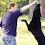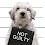## Thursday, February 21, 2013

### Wednesday Brain Teaser 2-20-13

I am thinking of a number between 1 and 1300.  It meets 3 criteria:

1. It is a perfect cube.
2. It is less than 500.
3. It is a perfect square.
Just kidding, it only meets two of those.  To make up for lying though, I'll tell you that it starts with 5, 7, or 9.

1.729 = 9^3 = 27^2

I got this by looking at cubes of numbers between 1 and 10. This was the second one that could be re-written as a square of some other number.

The first is 64 = 4^3 = 8^2, which would fit all 3 constraints. But doesn't fit the problem restatement...

No Googling.

2.3^2=9<500, satisfying conditions 2 and 3.

3.I didn't google. I wrote a quick pari+bash script to look for all the possibilities.

4.No googling when it's squares and cubes. Those and primes are the center of my numbers OCD. I used to factor things like the odometer for fun.

I had SJ's answer, but James's is much more elegant. I hazarded 512 as a guess because it was barely over 500, and I thought that might be the trick. But it didn't work, of course, because it's 2^9, and thus not a square. But figuring that cued me immediately that 9^3 was going to switch that and had to work.

But of course, 9 actually does begin with 9.

5.SJ definitely got the answer I was looking for, but James answer was quite lovely. Not sure how I missed that one, but I'm glad to be corrected!